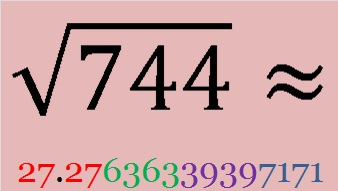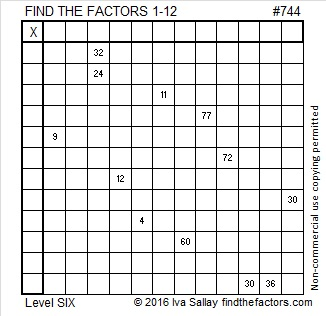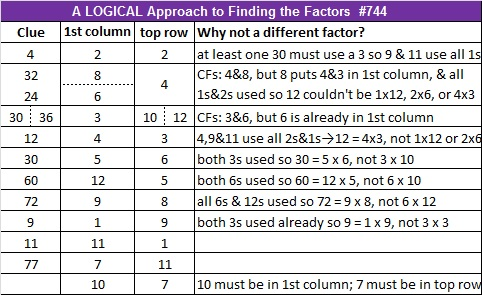# 744 Taiwan elects its first female president!

• 744 is a composite number.
• Prime factorization: 744 = 2 x 2 x 2 x 3 x 31, which can be written 744 = (2^3) x 3 x 31
• The exponents in the prime factorization are 3, 1, and 1. Adding one to each and multiplying we get (3 + 1)(1 + 1)(1 + 1) = 4 x 2 x 2 = 16. Therefore 744 has exactly 16 factors.
• Factors of 744: 1, 2, 3, 4, 6, 8, 12, 24, 31, 62, 93, 124, 186, 248, 372, 744
• Factor pairs: 744 = 1 x 744, 2 x 372, 3 x 248, 4 x 186, 6 x 124, 8 x 93, 12 x 62, or 24 x 31
• Taking the factor pair with the largest square number factor, we get √744 = (√4)(√186) = 2√186 ≈ 27.27636339397171.Today the majority in Taiwan defied mainland China and affirmed their belief in democracy as they overwhelmingly elected their first female president, Tsai Ing-wen. CNN reported that “her supporters filled streets, waving party banners and cheering to victory announcements made from a stage.”Taiwan’s first female president, Tsai Ing-wen; Source CNN

Apparently another way they celebrated was sharing my 689 post on Facebook over 4000 times and viewing that post 8346 times today. Since it was written, that post has been shared on Facebook over 7000 times, and today I recorded a record high 9017 views on my blog with 6289 views from Taiwan, 1122 from Hong Kong, 844 from the United States, and 33 views from China.

The Hong Kong Free Press gives a complete and up-to-date explanation in English of why 689 is the magic number and why so many people are interested in it. The 689 Coincidence explains it in Chinese.

Update: As mentioned in the comments, Tsai Ing-wen was elected with 6894744 votes. That’s an amazing coincidence! The election results not only repeated the number 689 but its last three digits are the same number as this post, 744. I now give some fun facts about that number:

744 has 16 factors, and the first 16 digits in its square root are 27.27636339397171Here is today’s puzzle. A logical way to find its solution is given in the table at the end of the post.Print the puzzles or type the solution on this excel file: 12 Factors 2016-01-11

Here’s a little more about the number 744:

744 can be written as the sum of consecutive numbers three different ways:

• 247 + 248 + 249 = 744; that’s 3 consecutive numbers.
• 39 + 40 + 41 + 42 + 43 + 44 + 45 + 46 + 47 + 48 + 49 + 50 + 51 + 52 + 53 + 54 = 744; that’s 16 consecutive numbers.
• 9 + 10 + 11 + 12 + 13 + 14 + 15 + 16 + 17 + 18 + 19 + 20 + 21 + 22 + 23 + 24 + 25 + 26 + 27 + 28 + 29 + 30 + 31 + 32 + 33 + 34 + 35 + 36 + 37 + 38 + 39 = 744; that’s 31 consecutive numbers.

744 is also the sum of 4 consecutive prime numbers: 179 + 181 + 191 + 193 = 744.

744 can also be written as the sum of three square numbers three different ways:

• 26² + 8² + 2² = 744
• 22² + 16² + 2² = 744
• 22² + 14² + 8² = 744

Notice that the squares of 8, 2, and 22 were each used two different times in those sums of squares. 26, 16, and 14 were each used once.

744 is also a palindrome in a couple other bases:

• 2112 BASE 7; note that 2(7^3) + 1(7^2) + 1(7^1) + 2(7^0) = 744.
• OO BASE 30 (O = 24); note that 24(30) + 24(1) = 744.

————————————-## 3 thoughts on “744 Taiwan elects its first female president!”

1.Arthur Lee

At a first glance, I thought you use the last 3 digits of number of votes for Tsai, which is 744, for the content of this post. But then, I note that it seems to be the number of puzzles you have made so far. Is it a coincidence?

•ivasallay

It’s a coincidence that amazes me. Thank you for informing me of it. I’ve updated the post with this correlation. Perhaps 744 can become her nickname if she doesn’t become the new 689.

The puzzle has the number 744 because this is my 744th post. Almost all of my posts have puzzles on them, but not all. Level 6 puzzles are difficult, but I hope you will give it a try. Today or tomorrow I’ll update the post with an order to look at the clues to find the puzzle’s solution logically.

2.Joseph Nebus

Well, wow. I should have started blogging about numbers long ago.

This site uses Akismet to reduce spam. Learn how your comment data is processed.